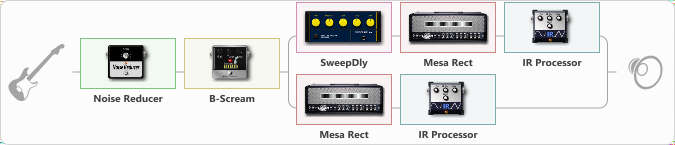# Metallica - puppets (redoubled)

Discussion in 'ToneLib-GFX presets' started by BGelais, Dec 14, 2022.

1. Metallica - puppets (redoubled)

Preset name: Metallica puppets

Effects chain:Effect: "Noise Reducer" (Dynamics / Filter), active - "yes"
{
"Sens" = 74
"Mode" = Soft
}

Effect: "B-Scream" (Overdrive / Distortion), active - "yes"
{
"Drive" = 0
"Tone" = 80
"Level" = 80
}

Effect: "Splitter" (Dynamics / Filter)
{
"A-Bypass" = Off
"A-Pan" = -100
"A-Level" = 50
"B-Bypass" = Off
"B-Pan" = 100
"B-Level" = 50
"Width" = 100

'A' branch:
{

Effect: "SweepDly" (Delay), active - "yes"
{
"Time" = 26
"Feedback" = 0
"Tone" = 70
"Sens" = 6
"Mix" = 100
}

Effect: "Mesa Rect" (Amp simulators), active - "yes"
{
"Gain" = 50
"Bass" = 100
"Middle" = 20
"Treble" = 50
"Presence" = 0
"Master" = 50
"Level (dB)" = 0
}

Effect: "IR Processor" (Cabinets), active - "yes"
{
"IR" = Puppet_IR
"Low Cut (Hz)" = 0
"Hi Cut (kHz)" = 10.5
"Mix" = 100
"Level (dB)" = 0
}
}
'B' branch:
{

Effect: "Mesa Rect" (Amp simulators), active - "yes"
{
"Gain" = 50
"Bass" = 100
"Middle" = 20
"Treble" = 50
"Presence" = 0
"Master" = 50
"Level (dB)" = 0
}

Effect: "IR Processor" (Cabinets), active - "yes"
{
"IR" = Puppet_IR
"Low Cut (Hz)" = 0
"Hi Cut (kHz)" = 10.0
"Mix" = 100
"Level (dB)" = 0
}
}
}

Note: You will need to download and install the ToneLib-GFX software to use the preset.

File size:
5.8 KB
Views:
3,512